Sample Paper Physics

# Physics Unsolved Sample Paper Solutions ICSE Class 10

## Section-A

(i) (c) 1 gf × cm = 980 dyne cm

(ii) (c) Turning the toothed wheel of a bicycle

(iii) (c) 1.25 × 108 ms–1

(iv) (a) Displacement and force are in same direction

(v) (d) Work done by both of them is different

(vi) (d) Both (a) and (c)

Explanation :

In damped vibration the amplitude reduces with time due to some external resistive force. Thus it is both forced and damped vibration.

(vii) (c) D

Explanation :

The vibrations produced in pendulum A will act as forced vibration to other pendulums through the rubber string XY. The pendulums B and C will remain in the state of forced vibrations while the pendulum D will come in the state of resonance. Because the natural frequency of pendulum D is equal to that of pendulum A (both pendulums A and D have the same length), energy will only be exchanged between the pendulums A and D. As a result, resonance will occur in pendulum D only.

(viii) (d) It is possible only if the truck’s running velocity is such that vibration frequency of some parts matches with the natural frequency of the piston.

Explanation :

Resonance will occur only if the truck’s running velocity is such that vibration frequency of some parts matches with the natural frequency of the piston.

(ix) (b) Trend C

Explanation :

Whether the resistances are connected in series or parallel. It will be a straight line. So trend C will not appear.

(x) (c) 10–15 to 10–14 m
Explanation :
The nucleus of an atom can be found at the centre of an atom whose size is of the order of 10–15 to 10–14 m and it consists of protons and neutrons where both proton and neutron are charged particles.

(xi) (c) Bulb C

Explanation :

As bulbs are in parallel connection, the only thing that will determine accessible current is resistances of the bulb. As bulb C has highest resistance, it will carry less current.

(xii) (a) 1:9

Explanation :
Heat produced, (H) = I2Rt. Here R and t are constant. Thus, HX/HY = (IX/IY)2 = 1/9. Thus the ratio of heat produced (HX:HY) = 1:9.

(xiii) (b) Light energy and heat energy

Explanation :

In electric lamp, electrical energy gets converted into light energy and heat energy. The bulb is heated, as a result of this heat energy.

(xiv) (c) Combination of neutrons and protons.

Explanation :

The nucleons are the major constituents of the nucleus, which are protons and neutrons. The total number of nucleons in the nucleus is referred to as the element’s mass number, and it is represented by the letter A.

(xv) (b) Concentric circle

Explanation :

Magnetic field lines around a straight current carrying conductor are in the form of concentric circle.

(i) Given : V = 30000 litre = 30 m3, m = Vρ = 30 × 103 kg, h = 45 m, t = 10 min. = 600 s, g = 9.8 ms–2
Work done by machine, W = mgh = (30 × 103) × 9.8 × 45
= 1.323 × 107 J

Power= W/t =1.323×107/600

= 2.205 × 104 W.

(ii) (a) One kilowatt hour is defined as the amount of electric energy consumed in an electrical circuit when an electric appliance of power 1 kW works for an hour.

1 kWh = 1000 W × 3600 s

= 1000 Js–1 × 3600 s
= 36 × 105 J = 3.6 × 106 J

(b) When a force F is applied at an angle θ to the direction of displacement s, then work done is given by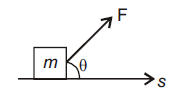W = Fs cos θ

(iii) (a) The completed ray diagram is shown below :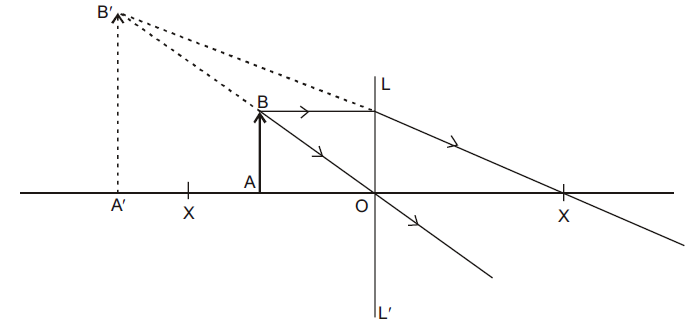(b) The lens LL′ is convex.

(c) Simple microscope.

(iv) In medical field, echo method of ultrasonic waves is used for imaging the human organs such as the liver, gall bladder, uterus etc.

(v) No, he cannot hear a clear echo, because the distance between the man and reflector should be 17 m.

(vi) Two 10 Ω resistances are connected in series,

R = 10 + 10 = 20 Ω

and 5 Ω resistance is connected in parallel to it,

R′ = 20 ×5/ 20+ 5
=100 /25
=4 Ω

Now, 3 Ω, R′ and 2 Ω are connected in series.
Hence,

R′′ = 3 + 4 + 2 = 9 Ω

(vii) Two ways by which the magnetic field of a solenoid can be made stronger are :

(a) Increasing the number of turns of the coil.
(b) Incresing the area of cross-section of the coil.

(i) Device used is total reflecting prism. It is used in prism binoculars.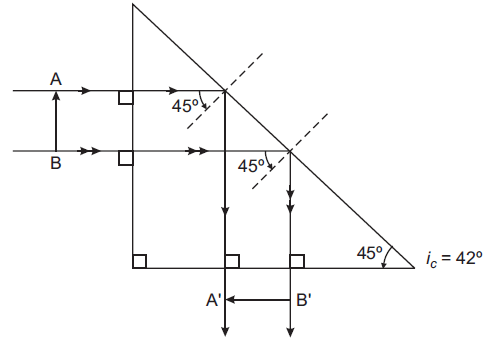(ii) (a) Object must be placed at 2F for the image to be of the same size.
(b) Object must be placed at F for the image to be at infinity.
(c) Object must be placed between F and 2F for the image to be inverted and enlarged.

(iii) (a) Emission of an a-particle :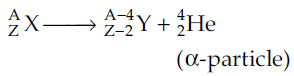The resulting nucleus has 2 protons and 2 neutrons (total 4 nucleons) less than the original (or parent) nucleus.
E.g.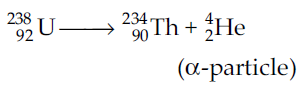(b) Emission of b-particle :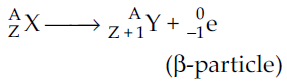The resulting nucleus has same number of nucleons. In this mass number remains same but atomic number increased by one.
E.g.(c) Gamma Emission :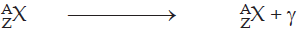There is no change in mass number (A) and atomic number (Z) of the nucleus in gamma emission.

(iv)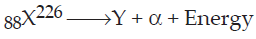(a) Atomic number of Y is 86 and mass number is 222.
(b) The gas formed is Helium (He)
(c) When the a-particle passes through a region containing a magnetic field, it gets deflected.

(v) (a) Heat capacity of lead piece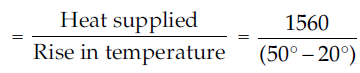= 52 J/°C

(b) Specific heat capacity of lead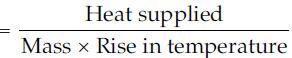= 0.13 J/g°C

## Section-B

(i) The S.I. unit of energy is Joule (J) and C.G.S. unit is erg.

1J = 107 erg.

(ii) (a) For an ideal machine, M.A. = V.R.
(b) (1) Nut cracker, (2) Single fixed pulley.

(iii) Given : m = 40 kg, g = 10 ms–2, S = 80×25/100 = 20 m, t = 25 s, L = 20 kgf = 20 × 10 = 200 N

(a) Gravitational force acting on the girl = mg = 40 × 10 = 400 N
(b) Total gravitational force (F) = 400 N + Load = 400 + 200 = 600 N
Work done by her = F × S = 600 × 20 = 12000 J
(c) Useful work done by her = Load × g × Displacement (or height)
= 20 × 10 × 20 = 4000 J

(d)  Power = Total work done/Time taken
= 12000/25

= 480 W.

(i) The complete figure is given below :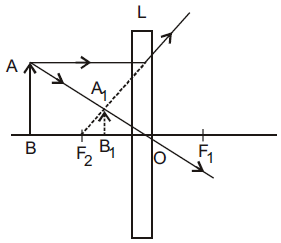The lens used is a concave lens.
The image formed is virtual, erect and diminished.

(ii) (a) AO = Real depth , AI = Apparent depth.(b) Refractive index of water (aμw) = Real depth (AO)/Apparent depth (AI)

(iii)(i) (a) Infrared radiations are used for photography in fog because they are not scattered by fog and can penetrate appreciably through it.

(b) Rock salt prism is used to obtain the spectrum of infrared radiations because a glass prism absorbs infrared radiations whereas a rock salt prism does not.

(ii) (a) The phenomenon is called resonance.

(b) This happens when the frequency of vibration of the piston of engine becomes equal to the natural frequency of vibration of the frame of motor cycle along with the rear view mirror.

(iii) (a) The diagram shows damped vibrations.

(b) A tuning fork vibrating in air.

(c) The amplitude of the wave decreases due to frictional force which the surrounding medium exerts on the vibrating body.

(d) After sometime the amplitude gradually decreases and finally stops.

(i) Three factors on which a current induced in a conductor placed in a magnetic field depends are :
(a) Number of turns of the conductor. Greater the number of turns more is the current in it.
(b) Area of cross section of the conductor. Greater the area of cross section of the conductor more is the current induced in it.
(c) Rate of change of magnetic flux linked with the conductor. Greater the magnetic lines of flux cut by the motion of a conductor more is the current induced in it.

(ii) (a) Superconductors are substances with zero resistance at very low temperatures.

(b) P = 110 W, V = 220 volt

Current

I = P /V =(110 /220)A = 0.5 A

(c) For semiconductors such as silicon, germanium, resistance decreases with the increase in temperature.

(iii) (a) Equivalent resistance of 3Ω and 1.5Ω in parallel,

R1= (3 ×1.5 /1 3 + 1.5) Ω =1Ω

Potential difference, across R1
⇒ V1 = IR1

= 0.3 × 1 = 0.3V

Current through 3W resistor,

I1 = V1/3=0.3/3=0.1A

(b) Total external resistance of the circuit, R = 1 + 4 = 5Ω

E = I (R + r)

⇒ 1.8 = 0.3 (5 + r)

⇒ 1.8/ 0.3 = 5 + r

⇒ 6 = 5 + r

(i) (a) Heat capacity of a body is the amount of heat energy required to raise its temperature by 1 Kelvin.

(b) SI Unit of heat capacity is joule per Kelvin (JK–1).

(c) Heat capacity = Mass × Specific heat capacity

(ii) (a) The slope of CD is less than slope of AB because specific heat capacity of liquid phase of same material can be different from that of solid phase of same material.

(b) Boiling point is t2°C and melting point is t1°C.

(iii) Let final temperature of water = x°C

Ice

m1 = 60 g
T1 = 0°C
T2 = x°C

Rise in temp.

(ΔT) = (x – 0)°C = x°C

Water

m2 = 140 g
T1 = 50°C
T2 = x°C

fall in temp.

(ΔT) = (50 – x)°C

Heat gained by ice = m1L + m1cΔT

= (60 × 336 + 60 × 4.2 × x) J

Heat lost by water = m2cΔT
Heat gained by ice = m1L + m1cDT

= 140 × 4.2 × (50 – x) J

Applying, the principle of mixtures,

140 × 4.2 × (50 – x) = 60 × 336 + 60 × 4.2 × x

⇒ 4.2 (7000 – 140x – 60x) = 60 × 336

7000 – 200x =(60×336)/4.2=(60×336×10)/42=4800

200x=2200

x = 11°C

Final temperature of water = 11°C

(i) (a) Particle emitted is β-particle.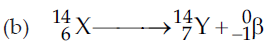(c) There will be no change in the nature of its radioactivity, because the phenomenon of radioactivity is not due to the orbital electrons which would be easily affected by such changes. Radioactivity is a nuclear phenomenon.

(ii) (a) This nuclear decay is allowed, since the atomic number and mass number are conserved.
(b) This nuclear decay is not allowed since the mass number is not conserved.

(c) This nuclear decay is not allowed since the atomic number and mass number both are not conserved.

(iii)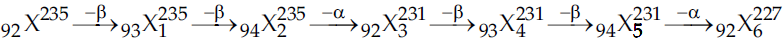The mass number and atomic number of X6 is 227 and 92 respectively#### ICSE 61 Sample Question Papers

All Subjects Combined for Class 10 Exam 2023

The dot mark field are mandatory, So please fill them in carefully
To download the Sample Paper (PDF File), Please fill & submit the form below.

Free Shipping on all
orderes over ₹ 1,000

Free 10-Day
Return Policy

15-Days Money-back
Guarantee

23,125 Products
Shipped this Month

Need Help?
+91-745 507 7222

Shopping cart